Atmos. Meas. Tech., 14, 3973–3988, 2021
https://doi.org/10.5194/amt-14-3973-2021
Atmos. Meas. Tech., 14, 3973–3988, 2021
https://doi.org/10.5194/amt-14-3973-2021

Research article 31 May 2021

Research article | 31 May 2021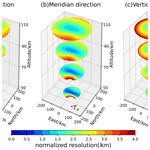# Error analyses of a multistatic meteor radar system to obtain a three-dimensional spatial-resolution distribution

Error analyses of a multistatic meteor radar system to obtain a three-dimensional spatial-resolution distribution
Wei Zhong1,2, Xianghui Xue1,2,3, Wen Yi1,2, Iain M. Reid4,5, Tingdi Chen1,2,3, and Xiankang Dou1,6 Wei Zhong et al.
• 1CAS Key Laboratory of Geospace Environment, Department of Geophysics and Planetary Sciences, University of Science and Technology of China, Hefei, China
• 2Mengcheng National Geophysical Observatory, School of Earth and Space Sciences, University of Science and Technology of China, Hefei, China
• 3CAS Center for Excellence in Comparative Planetology, Hefei, China
• 4ATRAD Pty Ltd., Thebarton, South Australia, Australia
• 5School of Physical Sciences, University of Adelaide, Adelaide, South Australia, Australia
• 6Electronic Information School, Wuhan University, Wuhan, China

Correspondence: Wei Zhong (zwei19@mail.ustc.edu.cn) and Wen Yi (yiwen@ustc.edu.cn)

Abstract

In recent years, the concept of multistatic meteor radar systems has attracted the attention of the atmospheric radar community, focusing on the mesosphere and lower thermosphere (MLT) region. Recently, there have been some notable experiments using such multistatic meteor radar systems. Good spatial resolution is vital for meteor radars because nearly all parameter inversion processes rely on the accurate location of the meteor trail specular point. It is timely then for a careful discussion focused on the error distribution of multistatic meteor radar systems. In this study, we discuss the measurement errors that affect the spatial resolution and obtain the spatial-resolution distribution in three-dimensional space for the first time. The spatial-resolution distribution can both help design a multistatic meteor radar system and improve the performance of existing radar systems. Moreover, the spatial-resolution distribution allows the accuracy of retrieved parameters such as the wind field to be determined.

Share
1 Introduction

The mesosphere and lower thermosphere (MLT) is a transition region from the neutral to the partially ionized atmosphere. It is dominated by the effects of atmospheric waves, including planetary waves, tides and gravity waves. It is also a relatively poorly sampled part of the Earth's atmosphere by ground-based instruments. One widely used approach to sample this region is the meteor radar technique. The ablation of incoming meteors in the MLT region, i.e.,  80–110 km, creates layers of metal atoms, which can be observed from the ground by photometry or lidar (Jia et al., 2016; Xue et al., 2013). During meteor ablation, the trails caused by small meteor particles provide a strong atmospheric tracer within the MLT region that can be continuously detected by meteor radars regardless of weather conditions. Consequently, the meteor radar technique has been a powerful tool for studying the MLT region for decades (Hocking et al., 2001; Holdsworth et al., 2004; Jacobi et al., 2008; Stober et al., 2013; Yi et al., 2018). Most modern meteor radars are monostatic, and this has two main limitations in retrieving the complete wind fields. Firstly, limited meteor rates and relatively low measurement accuracies necessitate that all measurements in the same height range are processed to calculate a “mean” wind. Secondly, classic monostatic radars retrieve winds based on the assumption of a homogenous wind in the horizontal and usually zero wind in the vertical direction.

The latter conditions can be partly relaxed if the count rates are high and the detections are distributed through a representative range of azimuths. If this is the case, a version of a velocity–azimuth display (VAD) analysis can be applied by expanding the zonal and meridional winds using a truncated Taylor expansion (Browning and Wexler, 1968). This is because each valid meteor detection yields a radial velocity in a particular viewing direction of the radar. The radar is effectively a multi-beam Doppler radar where the “beams” are determined by the meteor detections. If there are enough suitably distributed detections in the azimuth in a given observing period, the Taylor expansion approach (using Cartesian coordinates) yields the mean zonal and meridional wind components (u0,v0), the horizontal divergence $\left(\frac{\partial u}{\partial x}+\frac{\partial v}{\partial y}\right)$, and the stretching $\left(\frac{\partial u}{\partial x}-\frac{\partial v}{\partial y}\right)$ and shearing $\left(\frac{\partial u}{\partial y}+\frac{\partial v}{\partial x}\right)$ deformations of the wind fields from an analysis of the radial velocities. However, because the radar can only retrieve the wind projection in the radial direction as measured from the radar, the vorticity $\left(\frac{\partial v}{\partial x}-\frac{\partial u}{\partial y}\right)$ of the wind fields is not available. This is common to all monostatic radar systems, and a discussion of measurable parameters in the context of multiple fixed-beam upper-atmosphere Doppler radars is given by (Reid, 1987). Even when relaxing the assumption of a homogeneous wind fields and using the more advanced volume velocity processing (VVP) (Philippe and Corbin, 1979) to retrieve the wind fields, the horizontal gradients of the wind fields cannot be recovered due to the lack of vorticity information. To obtain a better understanding of the spatial variation of the MLT region wind fields, larger area observations (and hence higher meteor count rates) and sampling of the observed area from different viewing angles are needed. An extension of the classic monostatic meteor technique is required to satisfy these needs.

Analyzing spatial resolution limits is a fundamental but difficult topic for meteor radar systems. Meteor radar systems transmit and then receive radio waves reflected from meteor trails using a cluster of receiving antennas, commonly five antennas, as in the Jones et al. (1998) configuration. By analyzing the cross-correlations of the signals received on several pairs of antenna, the angle of arrival (AoA) of each return can be determined. The AoA is described by the zenith angle θ and azimuth angle ϕ. By measuring the wave propagation time from the meteor trail, range information can be determined. Most meteor radar systems rely on specular reflections from meteor trails. Thus, by combining the AoA and the range information and then using geometric analysis, the location of a meteor trail can be determined. Accurately locating the meteor trail specular point (MTSP hereinafter) is important since atmospheric parameter retrieval (such as the wind field or the temperature) depends on the location information of meteor trails. The location accuracy, namely the spatial resolution, determines the reliability of the retrieved parameters. For multistatic meteor radar systems that can relax the assumption of a homogenous horizontal wind field, the location accuracy becomes a more important issue because the horizontal spatial resolution affects the accuracy of the retrieved horizontal wind field.

Although meteor radar systems have developed well experimentally in recent years, the reliability of the retrieved atmospheric parameters still requires further investigation for both the monostatic and multistatic meteor radar cases. In an attempt to investigate errors in two radar techniques, Wilhelm et al. (2017) compared 11 years of MLT region wind data from a partial reflection (PR) radar with colocated monostatic meteor radar winds and determined the “correction factors” required to bring the winds into agreement. Spargo et al. (2019) reported a similar study for two locations for data obtained over several years. While the comparisons are interesting, partial reflection radars operating in the medium-frequency (MF) band and lower high-frequency (HF) band produce a height-dependent bias in the measured winds (see, e.g., Reid, 2015), which limits the ability to estimate errors in the meteor winds by comparing them. However, the PR radar technique is one of very few that provides day and night coverage and data rates in the MLT comparable to that of meteor radars.

Meteor radars have largely replaced PR radars for MLT studies and are generally regarded as providing reference-quality winds. It is essential then to know the reliability of atmospheric parameters determined by meteor radars, and to do this some quantitative error analyses are necessary.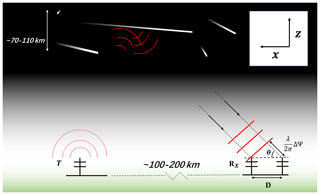Figure 1Schematic diagram of the simplified bistatic configuration used in Hocking's vertical resolution analysis (Hocking, 2018). The two receiving antennas and the transmitting antenna are collinear. The analysis is in a two-dimensional vertical section through the baseline joining the antennas. The radio wave is scattered from a few Fresnel zones that are several kilometers in length around the specular point on the meteor trail and are received by the receiving antennas. The cross-correlation analysis between the receiving antennas can be used to solve for the AoAs. Because the radio wave is reflected from a region a few Fresnel zones in length, the measured phase difference between the receiver antenna pairs to deviates from the ideal phase difference. This deviation from the ideal phase difference is one of the error sources in the PDME. In this work, we solve for the ideal phase difference associated with the AoA directed to the MTSP.

A number of recent studies have discussed AoA measurement errors for meteor radars (Kang, 2008; Vaudrin et al., 2018; Younger and Reid, 2017). These studies focus on the phase errors in receiver antenna pairs; see Younger and Reid (2017) for the monostatic case and Vaudrin et al. (2018) for a more general case that included multistatic meteor radars. Hocking (2018) used another approach and developed a vertical resolution analysis method for the two-dimensional bistatic case. The Hocking method (HM hereinafter) simplifies the error propagation process in the receiving antennas and puts emphasis on how a bistatic meteor radar configuration affects the vertical resolution in a vertical section. It does not consider the radial distance measuring error. In this paper, we consider the more general three-dimensional case and determine the spatial distribution of both the horizontal and vertical resolution uncertainties.

We analyze the multistatic meteor radar resolution distribution in a three-dimensional space for both vertical and horizontal resolution for the first time. This spatial resolution is a prerequisite for evaluating the reliability of retrieved atmospheric parameters, such as the wind field and the temperature.

2 Analytical method

## 2.1 Brief introduction

The HM will be introduced briefly here to help the reader understand our generalization. In the HM, measurement errors that affect the vertical resolution can be classified into two types: one caused by the zenith angle measuring error δθ and one caused by the pulse length effect on the vertical resolution. The receiving array is a simple antenna pair that is collinear with the baseline (Fig. 1). The HM calculates the vertical resolution in a two-dimensional vertical section that passes through the baseline. The receiver antenna pair is equivalent to one receiver arm in a Jones configuration that is comprised of three collinear antennas usually with a 2λ\2.5λ spacing. The phase difference of the received radio wave between the receiving antenna pair is denoted as ΔΨ. In meteor radar systems, there is generally an “acceptable” phase difference measuring error (PDME hereinafter) δ(ΔΨ). A higher value of δ(ΔΨ) means that more detected signals will be judged as meteor events but with more misidentifications and bigger errors as well. δ(ΔΨ) is set to approximately 30 (Hocking, 2018; Younger and Reid, 2017) in most meteor radar systems. In the HM, the zenith angle measuring error δθ is due to δ(ΔΨ) and δ(ΔΨ) is a constant. Therefore, the error propagation in the receiver is very simple, and δθ is inversely proportional to the cosine of the zenith angle.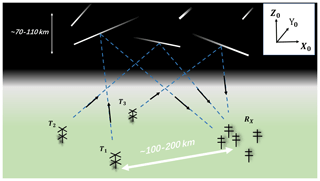Figure 2Schematic diagram of a multistatic meteor radar system using SIMO (single-input and multi-output). There are three transmitters (T1, T2 and T3) and one receiver (RX) in the picture. The transmitter–receiver distance is typically 100–200 km. X0, Y0 and Z0 represent the east, north and up directions of the receiving antenna. Over 90 % of the received energy comes from about 1 km around the specular point of the meteor trail, which is slightly less than the length of the central Fresnel zone (Ceplecha et al., 1998).

We now introduce our analytical method. Our method considers a multistatic system with multiple transmitters and one receiving array in three-dimensional space as shown in Fig. 2. The receiving array is in the Jones configuration, that is, “cross-shaped”, but it may also be “T-shaped” or “L-shaped”. The five receiver antennas are in the same horizontal plane and constitute two orthogonal antenna arms. To avoid a complex error propagation process in the receiving array and to place emphasis on multistatic configurations, the PDMEs in the two orthogonal antenna arms (δ(ΔΨ1) and δ(ΔΨ2)) are constants. Therefore, the AoA measuring errors (including the zenith and azimuth angle measuring errors δθ, δϕ, respectively) can be expressed as simple functions of zenith and azimuth angle. The radial distance is the distance between the MTSP and the receiver, which is denoted as Rs. Rs can be determined by combining the AoA, baseline length di and the radio wave propagation path length R (Stober and Chau, 2015). The geometry is shown in Fig. 4a. α is the angle between the baseline (i.e.,Xi axis) and the line from the receiver to the MTSP (denoted as point A). If α, di and R are known, Rs can be calculated easily using the cosine law as follows:

$\begin{array}{}\text{(1)}& {R}_{\mathrm{s}}=\frac{{R}^{\mathrm{2}}-{d}_{i}^{\mathrm{2}}}{\mathrm{2}\left(R-{d}_{i}\mathrm{cos}\mathit{\alpha }\right)}.\end{array}$

A multistatic configuration will influence the accuracy of Rs (denoted as δRs). This is because α, d and R are determined by the multistatic configuration. We consider the error term δRs in our method, which is ignored in the HM. δRs is a function of the AoA measuring errors (δθ and δϕ) and the radio wave propagation path length measuring error (denoted as δR). δR is caused by the measuring error of the wave propagation time δt, which is approximately 21 µs (Kang, 2008). Thus, δR can be set as a constant and the default value in our program is $\mathit{\delta }R=c\mathit{\delta }t=\mathrm{6.3}\phantom{\rule{0.125em}{0ex}}\mathrm{km}$. It is worth noting that the maximum unambiguous range for pulse meteor radars is determined by the pulse repetition frequency (PRF) (Hocking et al., 2001; Holdsworth et al., 2004). For multistatic meteor radars utilizing forward scatter, the maximum unambiguous range is $c/\text{PRF}$ (where c is the speed of light). For the area where R exceeds the maximum unambiguous range, δR is set to positive infinity.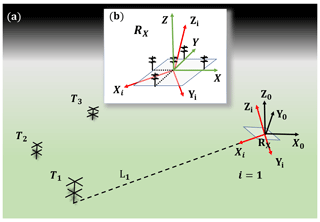Figure 3(a) Schematic diagram of the three coordinate systems used in this work. XiYiZi is a class of coordinate systems whose Xi axis points to transmitter i, with $i=\mathrm{1},\mathrm{2},\mathrm{3}$. X0Y0Z0 is the ENU coordinate system to which all errors are compared. (b) Magnified plot of the receiving array. XYZ is fixed on the receiver horizontal plane. The x and y axes are collinear with the two arms of the antenna array.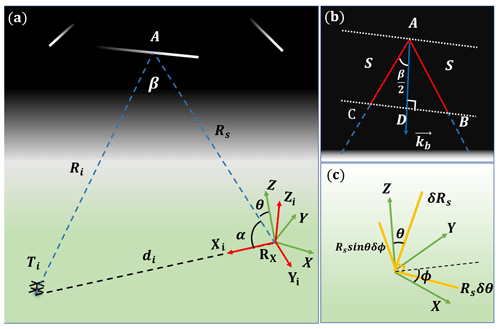Figure 4(a) Schematic diagram of the forward-scatter geometry for the radar link between Ti and RX. Point A is the MTSP. (b) Magnified plot of specular point A. The red line represents a radio wave pulse, and S is the half-pulse length. kb is the Bragg vector, which halves the forward-scatter angle β. (c) Schematic diagram of E1 in XYZ, which can be decomposed into three orthogonal vectors.

## 2.2 Three kinds of coordinate systems and their transformations

To better depict the multistatic system configuration, three kinds of right-hand coordinate systems need to be established, as shown in Fig. 3. These are X0Y0Z0, XiYiZi and XYZ. X0Y0Z0 is the ENU (east–north–up) coordinate system where the X0, Y0 and Z0 axes represent the east, north and up directions, respectively. Another two coordinate systems are established to facilitate different error propagations. All types of errors need to be transformed to the ENU coordinate system X0Y0Z0 in the end. Coordinate system XYZ is established to depict the spatial configuration of the receiving array and has its the origin of XYZ there, as shown in Fig. 3. The z axis is collinear with the antenna boresight and perpendicular to the horizontal plane on which the receiving array lies. The x and y axes are collinear with the arms of the two orthogonal antenna arrays. AoAs will be represented in XYZ for convenience. Inspection of Fig. 4 indicates that it is convenient to analyze the range information in a plane that goes through the baseline and the MTSP. Thus, a coordinate system XiYiZi is established for a transmitter Ti. The coordinate origins of XiYiZi are all on the receiving array. We stipulate that the Xi axis points to transmitter i (Ti). Each pair Ti and receiver RX constitute a radar link, which is referred to as Li. The range-related information for each Li will be calculated in XiYiZi. Different types of errors need to propagate to and be compared in X0Y0Z0, which is convenient for retrieving wind fields.

We stipulate that clockwise rotation satisfies the right-hand corkscrew rule. By rotating ${\mathit{\psi }}_{x}^{X,i}$, ${\mathit{\psi }}_{y}^{Y,i}$ and ${\mathit{\psi }}_{z}^{Z,i}$ about the x, y and z axes, respectively, one can transform XYZ to XiYiZi. It is worth mentioning that XiYiZi is non-unique because any rotation about Xi axis can obtain another satisfactory XiYiZi. Hence, ${\mathit{\psi }}_{x}^{X,i}$ can be set to any value. Similarly, by rotating ${\mathit{\psi }}_{x}^{\mathrm{i},\mathrm{0}}$, ${\mathit{\psi }}_{y}^{i,\mathrm{0}}$ and ${\mathit{\psi }}_{z}^{i,\mathrm{0}}$ about the x, y and z axes, respectively, one can transform XiYiZi to X0Y0Z0. To realize the coordinate transformation between these three coordinate systems, a coordinate rotation matrix ${\mathbf{A}}_{\mathbf{R}}\left({\mathit{\psi }}_{x},{\mathit{\psi }}_{y},{\mathit{\psi }}_{z}\right)$ is introduced. Using AR, one can transform the coordinate point or vector presentation from one coordinate system to another. The details of the coordinate rotation matrix ${\mathbf{A}}_{\mathbf{R}}\left({\mathit{\psi }}_{x},{\mathit{\psi }}_{y},{\mathit{\psi }}_{z}\right)$ can be found in Appendix A.

## 2.3 Two types of measuring errors

The analytical method of the spatial resolution for each radar link is the same. The difference between these radar links is only the value of the six coordinate rotation angles (${\mathit{\psi }}_{x}^{X,i}$, ${\mathit{\psi }}_{y}^{Y,i}$ and ${\mathit{\psi }}_{z}^{Z,i};{\mathit{\psi }}_{x}^{i,\mathrm{0}}$, ${\mathit{\psi }}_{y}^{i,\mathrm{0}}$ and ${\mathit{\psi }}_{z}^{i,\mathrm{0}}$) and the baseline distance di. The spatial-resolution-related measurement errors that will cause location errors of the MTSP can be classified into two types: E1 is caused by measurement errors at the receiver, and E2 is due to the pulse length. These two errors are mutually independent. Hence, the total error (Etotal) can be expressed as follows:

$\begin{array}{}\text{(2)}& {E}_{\text{total}}^{\mathrm{2}}={E}_{\mathrm{1}}^{\mathrm{2}}+{E}_{\mathrm{2}}^{\mathrm{2}}.\end{array}$

E1 is related to three indirect measuring errors. They are zenith, azimuth and radial distance measuring errors, denoted as δθ, δϕ and δRs, respectively. In XYZ, E1 can be decomposed into three orthogonal error vectors using δθ, δϕ and δRs (see Fig. 4c), which we now explain in more detail. PDMEs, i.e., δ(ΔΨ1) and δ(ΔΨ2), are caused by some practical factors, such as phase calibration mismatch and the fact that the specular point is not actually a point but is a few Fresnel zones in length. A meteor radar system calculates phase differences between different pairs of antenna through cross-correlation and then fits them to get the most likely AoAs. Therefore, the system needs to be assigned a tolerance value of δ(ΔΨ1) and δ(ΔΨ2). Different meteor radar systems have different AoA fit algorithms and thus different AoA measuring error distributions. To analyze the spatial resolution for a SIMO meteor radar system as generally as possible and to avoid tedious error propagation at the receiving array, we start the error propagation from δ(ΔΨ1) and δ(ΔΨ2) and set them as constants. AoA measuring errors δθ and δϕ can then be expressed as follows:

$\begin{array}{}\text{(3)}& & \mathit{\delta }\mathit{\theta }=\frac{\mathit{\lambda }}{\mathrm{2}\mathit{\pi }{D}_{\mathrm{1}}}\frac{\mathrm{cos}\mathit{\varphi }}{\mathrm{cos}\mathit{\theta }}\mathit{\delta }\left(\mathrm{\Delta }{\mathrm{\Psi }}_{\mathrm{1}}\right)+\frac{\mathit{\lambda }}{\mathrm{2}\mathit{\pi }{D}_{\mathrm{2}}}\frac{\mathrm{sin}\mathit{\varphi }}{\mathrm{cos}\mathit{\theta }}\mathit{\delta }\left(\mathrm{\Delta }{\mathrm{\Psi }}_{\mathrm{2}}\right),\text{(4)}& & \mathit{\delta }\mathit{\varphi }=\frac{\mathit{\lambda }}{\mathrm{2}\mathit{\pi }{D}_{\mathrm{2}}}\frac{\mathrm{cos}\mathit{\varphi }}{\mathrm{sin}\mathit{\theta }}\mathit{\delta }\left(\mathrm{\Delta }{\mathrm{\Psi }}_{\mathrm{2}}\right)-\frac{\mathit{\lambda }}{\mathrm{2}\mathit{\pi }{D}_{\mathrm{1}}}\frac{\mathrm{sin}\mathit{\varphi }}{\mathrm{sin}\mathit{\theta }}\mathit{\delta }\left(\mathrm{\Delta }{\mathrm{\Psi }}_{\mathrm{1}}\right),\end{array}$

where λ is the radio wavelength, D1 and D2 are the length of the two orthogonal antenna arms, and θ and ϕ are the zenith angle and the azimuth angle, respectively. The details can be found in Appendix A. It is worth noting that δθ and δϕ are not mutually independent. The expectation value of their product is not identical to zero unless $\frac{E\left({\mathit{\delta }}^{\mathrm{2}}\left(\mathrm{\Delta }{\mathrm{\Psi }}_{\mathrm{1}}\right)\right)}{{D}_{\mathrm{1}}^{\mathrm{2}}}$ is equal to $\frac{E\left({\mathit{\delta }}^{\mathrm{2}}\left(\mathrm{\Delta }{\mathrm{\Psi }}_{\mathrm{2}}\right)\right)}{{D}_{\mathrm{2}}^{\mathrm{2}}}$. δRs can be expressed as a function of δR, δθ and δϕ as follows:

$\begin{array}{}\text{(5)}& \begin{array}{rl}\mathit{\delta }{R}_{\mathrm{s}}& =F\left(\mathit{\delta }R,\mathit{\delta }\mathit{\theta },\mathit{\delta }\mathit{\varphi }\right)\\ & ={f}_{R}\left(\mathit{\theta },\mathit{\varphi }\right)\mathit{\delta }R+{f}_{\mathit{\theta }}\left(\mathit{\theta },\mathit{\varphi }\right)\mathit{\delta }\mathit{\theta }+{f}_{\mathit{\varphi }}\left(\mathit{\theta },\mathit{\varphi }\right)\mathit{\delta }\mathit{\varphi }.\end{array}\end{array}$

${f}_{R}\left(\mathit{\theta },\mathit{\varphi }\right),{f}_{\mathit{\theta }}\left(\mathit{\theta },\mathit{\varphi }\right)$ and fϕ(θ,ϕ) are the weighting functions of δRs. The details about the weighting function and deduction can be found in Appendix A. Inspection of Fig. 4c indicates that E1 can be decomposed into three orthogonal error vectors in coordinate XYZ, denoted as δRs, Rsδθ and Rssin θδϕ. These three vectors can be expressed in XYZ as follows:

$\begin{array}{}\text{(6)}& & \mathbit{\delta }{\mathbit{R}}_{\mathbf{s}}=\mathit{\delta }{R}_{\mathrm{s}}\left(\mathrm{sin}\mathit{\theta }\mathrm{cos}\mathit{\varphi },\mathrm{sin}\mathit{\theta }\mathrm{sin}\mathit{\varphi },\mathrm{cos}\mathit{\theta }{\right)}^{\mathrm{T}},\text{(7)}& & {\mathbit{R}}_{\mathbf{s}}\mathbit{\delta }\mathbit{\theta }={R}_{\mathrm{s}}\mathit{\delta }\mathit{\theta }\left(\mathrm{cos}\mathit{\theta }\mathrm{cos}\mathit{\varphi },\mathrm{cos}\mathit{\theta }\mathrm{sin}\mathit{\varphi },-\mathrm{sin}\mathit{\theta }{\right)}^{\mathrm{T}},\text{(8)}& & {\mathbit{R}}_{\mathbf{s}}\mathbf{sin}\mathbit{\theta }\mathbit{\delta }\mathbit{\varphi }={R}_{\mathrm{s}}\mathrm{sin}\mathit{\theta }\mathit{\delta }\mathit{\varphi }\left(-\mathrm{sin}\mathit{\varphi },\mathrm{cos}\mathit{\varphi },\mathrm{0}{\right)}^{\mathrm{T}}.\end{array}$

E2 is related to the radio wave propagation path. A pulse might be reflected anywhere within a pulse length (see Fig. 4b). This causes a location error in the MTSP, represented as an error vector DA. D is the median point of the isosceles triangle ΔABC's side BC. The representation of the error vector DA can be solved in XiYiZi by using geometrical relationships as follows:

$\begin{array}{}\text{(9)}& \begin{array}{rl}\mathbit{D}\mathbit{A}=\left(& \frac{\left(\mathrm{2}-{a}_{\mathrm{1}}-{a}_{\mathrm{2}}\right){x}_{i}+{d}_{i}\left({a}_{\mathrm{2}}-\mathrm{1}\right)}{\mathrm{2}},\frac{\left(\mathrm{2}-{a}_{\mathrm{1}}-{a}_{\mathrm{2}}\right){y}_{i}}{\mathrm{2}},\\ & \frac{\left(\mathrm{2}-{a}_{\mathrm{1}}-{a}_{\mathrm{2}}\right){z}_{i}}{\mathrm{2}}{\right)}^{\mathrm{T}},\end{array}\end{array}$

where S is the half-pulse length and ${a}_{\mathrm{1}}=\frac{{R}_{\mathrm{s}}-S}{{R}_{\mathrm{s}}}$. ${a}_{\mathrm{2}}=\frac{{R}_{i}-S}{{R}_{i}}$. di is the baseline length. $\left({x}_{i},{y}_{i},{z}_{i}\right)$ is the coordinate value of a MTSP (point A in Fig. 4) in XiYiZi. More details can be found in Appendix A.

## 2.4 Transformation to ENU coordinates

Thus far, two types of errors in different coordinate systems have been introduced. Now they need to be transformed to ENU coordinates X0Y0Z0 in order to compare different radar links and to analyze the wind fields. E1-related error vectors, which are three orthogonal vectors δRs, Rsδθ, and Rssin θδϕ, are represented in XYZ as Eqs. (6)–(8) and need to be transformed from XYZ to X0Y0Z0 to project δRs, Rsδθ and Rssin θδϕ towards the X0Y0Z0 axis, respectively, and reassemble them to form three new error vectors in the X0Y0Z0 axis. Using the coordinate rotation matrix ${{\mathbf{A}}_{\mathbf{R}}}^{\left(XYZ,{X}_{\mathrm{0}}{Y}_{\mathrm{0}}{Z}_{\mathrm{0}}\right)}={\mathbf{A}}_{\mathbf{R}}\left({\mathit{\psi }}_{x}^{i,\mathrm{0}},{\mathit{\psi }}_{y}^{i,\mathrm{0}},{\mathit{\psi }}_{z}^{i,\mathrm{0}}\right)\cdot {\mathbf{A}}_{\mathbf{R}}\left({\mathit{\psi }}_{x}^{X,i},{\mathit{\psi }}_{y}^{Y,i},{\mathit{\psi }}_{z}^{Z,i}\right)$ and Eqs. (6)–(8), the unit vectors of those three vectors can be represented in X0Y0Z0 as follows:

$\begin{array}{}\text{(10)}& \begin{array}{rl}& \left(\begin{array}{ccc}{\mathrm{X}}_{\mathrm{0}}^{\prime }\left(\mathit{\delta }{R}_{\mathrm{s}}\right)& {\mathrm{X}}_{\mathrm{0}}^{\prime }\left(\mathit{\delta }\mathit{\theta }\right)& {\mathrm{X}}_{\mathrm{0}}^{\prime }\left(\mathit{\delta }\mathit{\varphi }\right)\\ {\mathrm{Y}}_{\mathrm{0}}^{\prime }\left(\mathit{\delta }{R}_{\mathrm{s}}\right)& {\mathrm{Y}}_{\mathrm{0}}^{\prime }\left(\mathit{\delta }\mathit{\theta }\right)& {\mathrm{Y}}_{\mathrm{0}}^{\prime }\left(\mathit{\delta }\mathit{\varphi }\right)\\ {\mathrm{Z}}_{\mathrm{0}}^{\prime }\left(\mathit{\delta }{R}_{\mathrm{s}}\right)& {\mathrm{Z}}_{\mathrm{0}}^{\prime }\left(\mathit{\delta }\mathit{\theta }\right)& {\mathrm{Z}}_{\mathrm{0}}^{\prime }\left(\mathit{\delta }\mathit{\varphi }\right)\end{array}\right)={{\mathbf{A}}_{\mathbf{R}}}^{\left(XYZ,{X}_{\mathrm{0}}{Y}_{\mathrm{0}}{Z}_{\mathrm{0}}\right)}\\ & \phantom{\rule{0.25em}{0ex}}\phantom{\rule{0.25em}{0ex}}\phantom{\rule{0.25em}{0ex}}\phantom{\rule{0.25em}{0ex}}\cdot \left(\begin{array}{ccc}\mathrm{sin}\mathit{\theta }\mathrm{cos}\mathit{\varphi }& \mathrm{cos}\mathit{\theta }\mathrm{cos}\mathit{\varphi }& -\mathrm{sin}\mathit{\varphi }\\ \mathrm{sin}\mathit{\theta }\mathrm{sin}\mathit{\varphi }& \mathrm{cos}\mathit{\theta }\mathrm{sin}\mathit{\varphi }& \mathrm{cos}\mathit{\varphi }\\ \mathrm{cos}\mathit{\theta }& -\mathrm{sin}\mathit{\theta }& \mathrm{0}\end{array}\right).\end{array}\end{array}$

${\left({\mathbf{X}}_{\mathbf{0}}^{\mathbf{\prime }}\mathbf{\left(}\mathbit{\delta }{\mathbit{R}}_{\mathbf{s}}\mathbf{\right)}\mathbf{,}{\mathbit{Y}}_{\mathbf{0}}^{\mathbf{\prime }}\mathbf{\left(}\mathbit{\delta }{\mathbit{R}}_{\mathbf{s}}\mathbf{\right)}\mathbf{,}{\mathbit{Z}}_{\mathbf{0}}^{\mathbf{\prime }}\mathbf{\left(}\mathbit{\delta }{\mathbit{R}}_{\mathbf{s}}\mathbf{\right)}\right)}^{\mathbf{T}}$, ${\left({\mathbit{X}}_{\mathbf{0}}^{\mathbf{\prime }}\mathbf{\left(}\mathbit{\delta }\mathbit{\theta }\mathbf{\right)}\mathbf{,}{\mathbit{Y}}_{\mathbf{0}}^{\mathbf{\prime }}\mathbf{\left(}\mathbit{\delta }\mathbit{\theta }\mathbf{\right)}\mathbf{,}{\mathbit{Z}}_{\mathbf{0}}^{\mathbf{\prime }}\mathbf{\left(}\mathbit{\delta }\mathbit{\theta }\mathbf{\right)}\right)}^{\mathbf{T}}$, and ${\left({\mathbit{X}}_{\mathbf{0}}^{\mathbf{\prime }}\mathbf{\left(}\mathbit{\delta }\mathbit{\varphi }\mathbf{\right)}\mathbf{,}{\mathbit{Y}}_{\mathbf{0}}^{\mathbf{\prime }}\mathbf{\left(}\mathbit{\delta }\mathbit{\varphi }\mathbf{\right)}\mathbf{,}{\mathbit{Z}}_{\mathbf{0}}^{\mathbf{\prime }}\mathbf{\left(}\mathbit{\delta }\mathbit{\varphi }\mathbf{\right)}\right)}^{\mathbf{T}}$ are unit vectors of δRs, Rsδθ, and Rssin θδϕ in X0Y0Z0, respectively. The 3×3 matrix on the left-hand side of Eq. (10) is denoted as Pij for $i,j=\mathrm{1},\mathrm{2},\mathrm{3}$.

From Eqs. (6)–(8) and Fig. 4c, we see that the length of those three vectors (the error values) is δRs, Rsδθ, Rssin θδϕ as a function of δR, δθ, δϕ. In order to reassemble them to form new error vectors, transformation of δθ and δϕ into two independent errors δ(ΔΨ1) and δ(ΔΨ2) is needed because δθ and δϕ are not independent. Using Eqs. (3) and (4), one can transform vector ${\left(\mathit{\delta }R\phantom{\rule{0.125em}{0ex}},\phantom{\rule{0.125em}{0ex}}\phantom{\rule{0.125em}{0ex}}\mathit{\delta }\mathit{\theta }\phantom{\rule{0.125em}{0ex}},\phantom{\rule{0.125em}{0ex}}\phantom{\rule{0.125em}{0ex}}\mathit{\delta }\mathit{\varphi }\right)}^{\mathrm{T}}$ into three independent measuring errors δR, δ(ΔΨ1) and δ(ΔΨ2). Thus, ${\left(\mathit{\delta }{R}_{\mathrm{s}},{R}_{\mathrm{s}}\mathit{\delta }\mathit{\theta },{R}_{\mathrm{s}}\mathrm{sin}\mathit{\theta }\mathit{\delta }\mathit{\varphi }\right)}^{\mathrm{T}}$ can be expressed as follows:

$\begin{array}{}\text{(11)}& \begin{array}{rl}\left(\begin{array}{c}\mathit{\delta }{R}_{\mathrm{s}}\\ {R}_{\mathrm{s}}\mathit{\delta }\mathit{\theta }\\ {R}_{\mathrm{s}}\mathrm{sin}\mathit{\theta }\mathit{\delta }\mathit{\varphi }\end{array}\right)& =\left(\begin{array}{ccc}{f}_{R}\left(\mathit{\theta },\mathit{\varphi }\right)& {f}_{\mathit{\theta }}\left(\mathit{\theta },\mathit{\varphi }\right)& {f}_{\mathit{\varphi }}\left(\mathit{\theta },\mathit{\varphi }\right)\\ \mathrm{0}& {R}_{\mathrm{s}}& \mathrm{0}\\ \mathrm{0}& \mathrm{0}& {R}_{\mathrm{s}}\mathrm{sin}\mathit{\theta }\end{array}\right)\\ & \phantom{\rule{0.25em}{0ex}}\phantom{\rule{0.25em}{0ex}}\phantom{\rule{0.25em}{0ex}}\cdot \left(\begin{array}{ccc}\mathrm{1}& \mathrm{0}& \mathrm{0}\\ \mathrm{0}& \frac{\frac{\mathit{\lambda }}{\mathrm{2}\mathit{\pi }}}{\mathrm{cos}\mathit{\theta }}\frac{\mathrm{cos}\mathit{\varphi }}{{D}_{\mathrm{1}}}& -\frac{\frac{\mathit{\lambda }}{\mathrm{2}\mathit{\pi }}\mathrm{sin}\mathit{\varphi }}{\mathrm{sin}\mathit{\theta }{D}_{\mathrm{1}}}\\ \mathrm{0}& \frac{\frac{\mathit{\lambda }}{\mathrm{2}\mathit{\pi }}}{\mathrm{cos}\mathit{\theta }}\frac{\mathrm{sin}\mathit{\varphi }}{{D}_{\mathrm{2}}}& \frac{\frac{\mathit{\lambda }}{\mathrm{2}\mathit{\pi }}\mathrm{cos}\mathit{\varphi }}{\mathrm{sin}\mathit{\theta }{D}_{\mathrm{2}}}\end{array}\right)\\ & \phantom{\rule{0.25em}{0ex}}\phantom{\rule{0.25em}{0ex}}\phantom{\rule{0.25em}{0ex}}\cdot \left(\begin{array}{c}\mathit{\delta }R\\ \mathit{\delta }\left(\mathrm{\Delta }{\mathrm{\Psi }}_{\mathrm{1}}\right)\\ \mathit{\delta }\left(\mathrm{\Delta }{\mathrm{\Psi }}_{\mathrm{2}}\right)\end{array}\right).\end{array}\end{array}$

The product of the first and the second term on the right-hand side of Eq. (11) is a 3×3 matrix, denoted as Wij for $i,j=\mathrm{1},\mathrm{2},\mathrm{3}$. From Eq. (11), we see that the three error values δRs, Rsδθ and Rssin θδϕ are the linear combinations of δR, δ(ΔΨ1),and δ(ΔΨ2) with their corresponding linear coefficients W1j, W2j and W3j. Those three error values can be projected toward new directions (e.g., the X0Y0Z0 axis) by using Pij. It worth noting that in a new direction the same basis's projected linear coefficients from different error values should be used to calculate their sum of squares (SS). Following this, the square root of SS will be used as a new linear coefficient for that basis in the new direction. For example, in X0 directions, basis δ(ΔΨ1)'s projected linear coefficients are ${\mathrm{X}}_{\mathrm{0}}^{\prime }\left(\mathit{\delta }{R}_{\mathrm{s}}\right){W}_{\mathrm{12}}$, ${\mathrm{X}}_{\mathrm{0}}^{\prime }\left(\mathit{\delta }\mathit{\theta }\right){W}_{\mathrm{22}}$ and ${\mathrm{X}}_{\mathrm{0}}^{\prime }\left(\mathit{\delta }\mathit{\varphi }\right){W}_{\mathrm{32}}$ from δRs, Rsδθ and Rssin θδϕ, respectively. Therefore, the new linear coefficient for δ(ΔΨ1) in the X0 direction is ${W}_{{\mathrm{X}}_{\mathrm{0}}^{\prime }}^{\mathit{\delta }\left(\mathrm{\Delta }{\mathrm{\Psi }}_{\mathrm{1}}\right)}=±\sqrt{{\left({\mathrm{X}}_{\mathrm{0}}^{\prime }\left(\mathit{\delta }{R}_{\mathrm{s}}\right){W}_{\mathrm{12}}\right)}^{\mathrm{2}}+{\left({\mathrm{X}}_{\mathrm{0}}^{\prime }\left(\mathit{\delta }\mathit{\theta }\right){W}_{\mathrm{22}}\right)}^{\mathrm{2}}+{\left({\mathrm{X}}_{\mathrm{0}}^{\prime }\left(\mathit{\delta }\mathit{\varphi }\right){W}_{\mathrm{32}}\right)}^{\mathrm{2}}}$. Similarly, one can get δR and δ(ΔΨ2)'s new linear coefficients in ${\mathrm{X}}_{\mathrm{0}}^{\prime }$, denoted as ${W}_{{\mathrm{X}}_{\mathrm{0}}^{\prime }}^{\mathit{\delta }R}$ and ${W}_{{\mathrm{X}}_{\mathrm{0}}^{\prime }}^{\mathit{\delta }\left(\mathrm{\Delta }{\mathrm{\Psi }}_{\mathrm{2}}\right)}$. Thus, the true error value in the X0 direction is ${W}_{{\mathrm{X}}_{\mathrm{0}}^{\prime }}^{\mathit{\delta }R}\mathit{\delta }R+{W}_{{\mathrm{X}}_{\mathrm{0}}^{\prime }}^{\mathit{\delta }\left(\mathrm{\Delta }{\mathrm{\Psi }}_{\mathrm{1}}\right)}\mathit{\delta }\left(\mathrm{\Delta }{\mathrm{\Psi }}_{\mathrm{1}}\right)+{W}_{{\mathrm{X}}_{\mathrm{0}}^{\prime }}^{\mathit{\delta }\left(\mathrm{\Delta }{\mathrm{\Psi }}_{\mathrm{2}}\right)}\mathit{\delta }\left(\mathrm{\Delta }{\mathrm{\Psi }}_{\mathrm{2}}\right)$. Because δR, δ(ΔΨ1), and δ(ΔΨ2) are mutually independent, E1 is related to the mean squared error (MSE) values in the X0 direction, denoted as δ(1)X0 and can be expressed as ${\mathit{\delta }}_{\left(\mathrm{1}\right)}{X}_{\mathrm{0}}=±$ $\sqrt{{\left({W}_{{X}_{\mathrm{0}}^{\prime }}^{\mathit{\delta }R}\mathit{\delta }R\right)}^{\mathrm{2}}+{\left({W}_{{X}_{\mathrm{0}}^{\prime }}^{\mathit{\delta }\left(\mathrm{\Delta }{\mathrm{\Psi }}_{\mathrm{1}}\right)}\mathit{\delta }\left(\mathrm{\Delta }{\mathrm{\Psi }}_{\mathrm{1}}\right)\right)}^{\mathrm{2}}+{\left({W}_{{X}_{\mathrm{0}}^{\prime }}^{\mathit{\delta }\left(\mathrm{\Delta }{\mathrm{\Psi }}_{\mathrm{2}}\right)}\mathit{\delta }\left(\mathrm{\Delta }{\mathrm{\Psi }}_{\mathrm{2}}\right)\right)}^{\mathrm{2}}}$.

In short, E1-related errors in ENU coordinate's three axis directions (denoted as δ(1)X0, δ(1)Y0 and δ(1)Z0) can be expressed in the form of a matrix as follows:

$\begin{array}{}\text{(12)}& \left(\begin{array}{c}{\mathit{\delta }}_{\left(\mathrm{1}\right)}^{\mathrm{2}}{X}_{\mathrm{0}}\\ {\mathit{\delta }}_{\left(\mathrm{1}\right)}^{\mathrm{2}}{Y}_{\mathrm{0}}\\ {\mathit{\delta }}_{\left(\mathrm{1}\right)}^{\mathrm{2}}{Z}_{\mathrm{0}}\end{array}\right)={P}_{ij}^{\mathrm{2}}\cdot {W}_{ij}^{\mathrm{2}}\cdot \left(\begin{array}{c}{\mathit{\delta }}^{\mathrm{2}}R\\ {\mathit{\delta }}^{\mathrm{2}}\left(\mathrm{\Delta }{\mathrm{\Psi }}_{\mathrm{1}}\right)\\ {\mathit{\delta }}^{\mathrm{2}}\left(\mathrm{\Delta }{\mathrm{\Psi }}_{\mathrm{2}}\right)\end{array}\right).\end{array}$

The E2-related error vector DA needs transformation from XiYiZi to X0Y0Z0. Therefore, E2-related errors in the ENU coordinate's three axis directions (denoted as δ(2)X0, δ(2)Y0 and δ(2)Z0) can be expressed in the form of a matrix as follows:

$\begin{array}{}\text{(13)}& \left(\begin{array}{c}{\mathit{\delta }}_{\left(\mathrm{2}\right)}{X}_{\mathrm{0}}\\ {\mathit{\delta }}_{\left(\mathrm{2}\right)}{Y}_{\mathrm{0}}\\ {\mathit{\delta }}_{\left(\mathrm{2}\right)}{Z}_{\mathrm{0}}\end{array}\right)=±{\mathbf{A}}_{\mathbf{R}}\left({\mathit{\psi }}_{x}^{i,\mathrm{0}},{\mathit{\psi }}_{y}^{i,\mathrm{0}},{\mathit{\psi }}_{z}^{i,\mathrm{0}}\right)\cdot \mathbit{D}\mathbit{A}.\end{array}$

E1 and E2 are mutually independent. By using Eq. (1), the total MSE values in ENU coordinate's three axis directions (denoted as δtotalX0, δtotalY0 and δtotalZ0) can be expressed in the form of matrix as follows:

$\begin{array}{}\text{(14)}& \left(\begin{array}{c}{\mathit{\delta }}_{\text{total}}^{\mathrm{2}}{X}_{\mathrm{0}}\\ {\mathit{\delta }}_{\text{total}}^{\mathrm{2}}{Y}_{\mathrm{0}}\\ {\mathit{\delta }}_{\text{total}}^{\mathrm{2}}{Z}_{\mathrm{0}}\end{array}\right)=\left(\begin{array}{c}{\mathit{\delta }}_{\left(\mathrm{1}\right)}^{\mathrm{2}}{X}_{\mathrm{0}}\\ {\mathit{\delta }}_{\left(\mathrm{1}\right)}^{\mathrm{2}}{Y}_{\mathrm{0}}\\ {\mathit{\delta }}_{\left(\mathrm{1}\right)}^{\mathrm{2}}{Z}_{\mathrm{0}}\end{array}\right)+\left(\begin{array}{c}{\mathit{\delta }}_{\left(\mathrm{2}\right)}^{\mathrm{2}}{X}_{\mathrm{0}}\\ {\mathit{\delta }}_{\left(\mathrm{2}\right)}^{\mathrm{2}}{Y}_{\mathrm{0}}\\ {\mathit{\delta }}_{\left(\mathrm{2}\right)}^{\mathrm{2}}{Z}_{\mathrm{0}}\end{array}\right).\end{array}$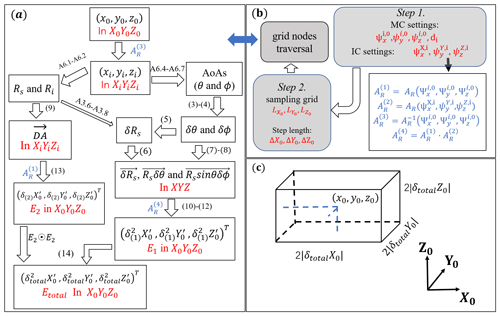Figure 5(a) The flow chart of the location error calculation process for a point in X0Y0Z0. The notation beside the arrows represents the corresponding equations (black) or coordinate rotation matrix (blue) in the paper.  is the Hadamard product. Thus, E2E2 will yield $\left({\mathit{\delta }}_{\left(\mathrm{2}\right)}^{\mathrm{2}}{X}_{\mathrm{0}},{\mathit{\delta }}_{\left(\mathrm{2}\right)}^{\mathrm{2}}{Y}_{\mathrm{0}},{\mathit{\delta }}_{\left(\mathrm{2}\right)}^{\mathrm{2}}{Z}_{\mathrm{0}}{\right)}^{\mathrm{T}}$. (b) The flow chart of the program to calculate the location errors distributions for a radar link Li. This process includes parameters settings for a radar link, the generation of the sampling grid nodes and the traversing of all the nodes. For each node, the program uses the calculation method described in (a). MC is the multistatic configuration, and IC is the interferometer (receiving antenna) configuration. (c) Schematic diagram of the relationship between the spatial resolution and the total location errors of the MTSP. For a detected point in space, the MSE of MTSP's location errors is ±|δtotalX0|, ±|δtotalY0| and ±|δtotalZ0| in the zonal, meridional and vertical directions, respectively. This means that the actual specular point might occur in a region that forms a $\mathrm{2}\mathrm{|}{\mathit{\delta }}_{\text{total}}{X}_{\mathrm{0}}\mathrm{|}×\mathrm{2}\mathrm{|}{\mathit{\delta }}_{\text{total}}{Y}_{\mathrm{0}}\mathrm{|}×\mathrm{2}\mathrm{|}{\mathit{\delta }}_{\text{total}}{Z}_{\mathrm{0}}\mathrm{|}$ cube and that the detected point is on the centroid of this cube.

In conclusion, for a radar link Li and a MTSP represented as $\left({x}_{\mathrm{0}},{y}_{\mathrm{0}},{z}_{\mathrm{0}}\right)$ in the ENU coordinate system X0Y0Z0, as sketched in Fig. 4a, the location errors of this point in east, north and up directions (±δtotalX0, ±δtotalY0 and ±δtotalZ0) can be calculated as follows: firstly, for a point $\left({x}_{\mathrm{0}},{y}_{\mathrm{0}},{z}_{\mathrm{0}}\right)$ in ${\mathrm{X}}_{\mathrm{0}}^{\prime }{Y}_{\mathrm{0}}^{\prime }{\mathrm{Z}}_{\mathrm{0}}^{\prime }$, use AR to transform it to XiYiZi and denote it as $\left({x}_{i},{y}_{i},{z}_{i}\right)$. Following this, in XiYiZi calculate the AoA (θ and ϕ) and the range information (Rs and Ri). Details of AoA and range calculation can be found in Appendix A. It is worth noting that the AoA is given by the angles relative to the axes of XYZ. Secondly, in XYZ use the AoA and Eqs. (3)–(8) to calculate E1's three orthogonal error vectors shown in Fig. 4c; in XiYiZi use the range information and Eq. (9) to calculate E2's error vector DA, as shown in Fig. 4b. Thirdly, project E1's three error vectors to X0Y0Z0 by using Eq. (10) and use Eqs. (11)–(12) to reassemble them to calculate E1-related MSE values in the direction of X0Y0Z0; use Eq. (13) to transform the E2 error vector from XiYiZi to X0Y0Z0. Finally, use Eq. (14) to get the total location errors of a MTSP in $\left({x}_{\mathrm{0}},{y}_{\mathrm{0}},{z}_{\mathrm{0}}\right)$. Figure 5a shows the flow chart for the process we have just described.

3 Results and discussion

The program to study the method we have described above is written in the Python language and is presented in the Supplement. To calculate a special configuration of a multistatic radar system, we initially need to set six coordinate transformation angles (${\mathit{\psi }}_{x}^{X,i}$, ${\mathit{\psi }}_{y}^{Y,i}$ and ${\mathit{\psi }}_{z}^{Z,i};{\mathit{\psi }}_{x}^{i,\mathrm{0}}$, ${\mathit{\psi }}_{y}^{i,\mathrm{0}}$ and ${\mathit{\psi }}_{z}^{i,\mathrm{0}}$) and the baseline length di for each radar link Li. For example, ${\mathit{\psi }}_{x}^{i,\mathrm{0}}={\mathit{\psi }}_{y}^{i,\mathrm{0}}=\mathrm{0}$, ${\mathit{\psi }}_{z}^{i,\mathrm{0}}=\mathrm{30}{}^{\circ }$ and di=250 km means that transmitter Ti is 250 km, 30 east by south of the receiver RX. Further, ${\mathit{\psi }}_{x}^{X,i}=\mathrm{5}$, ${\mathit{\psi }}_{y}^{Y,i}=\mathrm{0}$, ${\mathit{\psi }}_{z}^{Z,i}=\mathrm{0}$ means one receiver arm (y axis) points to 60 east by north with a 5 elevation. The detection area of interest for a multistatic meteor radar is usually from 70 to 110 km in height and around 300 km× 300 km in the horizontal. In our program, this area needs to be divided into a spatial grid for sampling. The default value of the sampling grid length is 1 km in height and 5 km in the meridional and zonal directions. After selecting the desired settings, the program steps though the sampling grid nodes and calculates the location errors at each node as described in Fig. 5a. Figure 5b describes the parameter settings and the transversal calculation process. For a given setting of radar link Li, the program will output the squared values of E1-related, E2-related and total MSE values (${E}_{\text{total}}^{\mathrm{2}}$: ${\mathit{\delta }}_{\text{total}}^{\mathrm{2}}{X}_{\mathrm{0}},{\mathit{\delta }}_{\text{total}}^{\mathrm{2}}{Y}_{\mathrm{0}},{\mathit{\delta }}_{\text{total}}^{\mathrm{2}}{Z}_{\mathrm{0}}$; ${E}_{\mathrm{1}}^{\mathrm{2}}$: ${\mathit{\delta }}_{\left(\mathrm{1}\right)}^{\mathrm{2}}{X}_{\mathrm{0}}$, ${\mathit{\delta }}_{\left(\mathrm{1}\right)}^{\mathrm{2}}{Y}_{\mathrm{0}},{\mathit{\delta }}_{\left(\mathrm{1}\right)}^{\mathrm{2}}{Z}_{\mathrm{0}}$; ${E}_{\mathrm{2}}^{\mathrm{2}}$: ${\mathit{\delta }}_{\left(\mathrm{2}\right)}^{\mathrm{2}}{X}_{\mathrm{0}},{\mathit{\delta }}_{\left(\mathrm{2}\right)}^{\mathrm{2}}{Y}_{\mathrm{0}},{\mathit{\delta }}_{\left(\mathrm{2}\right)}^{\mathrm{2}}{Z}_{\mathrm{0}}$). The location errors can be positive or negative, and thus the spatial resolutions are twice the absolute value of the location errors. For an example, see Fig. 5c. For a detected MTSP, represented as $\left({x}_{\mathrm{0}},{y}_{\mathrm{0}},{z}_{\mathrm{0}}\right)$ in X0Y0Z0, with ${\mathit{\delta }}_{\text{total}}^{\mathrm{2}}{X}_{\mathrm{0}},{\mathit{\delta }}_{\text{total}}^{\mathrm{2}}{Y}_{\mathrm{0}}$ and ${\mathit{\delta }}_{\text{total}}^{\mathrm{2}}{Z}_{\mathrm{0}}$ equal to 25, 16 and 9 km2, respectively, the actual position of the MTSP could occur in an area that is ± 5, ± 4 and ± 3 km around $\left({x}_{\mathrm{0}},{y}_{\mathrm{0}},{z}_{\mathrm{0}}\right)$ with equal probability. Consequently, the zonal, meridional and vertical resolutions are 10, 8 and 6 km, respectively.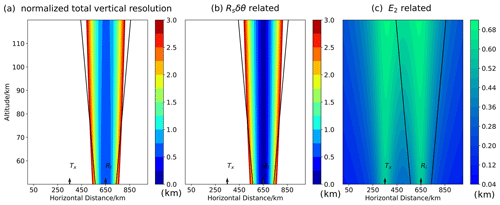Figure 6The normalized vertical resolution distribution in a vertical section from 50 to 120 km height when the error term “δRs” is ignored. Panels (a–c) are the total, Rsδθ-related and E2-related normalized resolution distributions, respectively. These results are the same as those produced in Hocking's work (Hocking, 2018). The two black arrows represent the positions right above the transmitter (Tx) and the receiver (Rx) and the transmitter–receiver separation is 300 km. The region between the two oblique black lines is the sampling volume for the receiving array because the elevation angle is beyond 30 to reduce influence from potential mutual antenna coupling or from other obstacles in the surrounding area. Except the region at large elevation angles (i.e., 90), the E2-related resolution values are much lower than the Rsδθ-related errors. The Rsδθ-related resolution distribution only depends on the receiving antennas. Thus, the total vertical resolution distribution is nearly unchanged with the variation of the transmitter–receiver distance. Normalized resolution values that exceed 3 km (which correspond 12 km vertical resolution) are not shown.

The HM analyzes the vertical resolution (corresponding to δZ0 in our paper) in a two-dimensional vertical section (corresponding to the X0Z0 plane in our paper). To compare with Hocking's work, ${\mathit{\psi }}_{z}^{i,\mathrm{0}}$ is set to 180, and the other five coordinate transformation angles are all set to zero with d equal to 300 km. The half-pulse length S is set to 2 km and δ(ΔΨ1) to 35. Calculating in the X0Y0 plane only should have degraded our method into Hocking's two-dimensional analysis method but does not because the HM method ignores δRs. In fact, the HM considers only E2 and Rsδθ in the X0Y0 plane. Consequently, we need to further set fR(θ,ϕ), fθ(θ,ϕ) and fϕ(θ,ϕ) to be zero. When this is done, our method degrades into the HM. Hocking's results are shown as the absolute value of vertical location error normalized relative to the half-pulse width $\mathrm{|}\mathit{\delta }{Z}_{\mathrm{0}}\mathrm{|}/S$. Hereinafter, $\mathrm{|}E\mathrm{|}/S$ is referred to as the normalized spatial resolution, such as δ(1)X0 and δtotalY0, where E represents the location errors in a direction. Thus, the spatial resolutions are 2S times the normalized spatial resolutions.

Our normalized vertical resolution distributions are shown in Fig. 6a and are the same as those presented in Hocking's work (Hocking, 2018). The distribution of Rsδθ-related, E2-related and total normalized vertical resolution distributions are shown in Fig. 6 from left to right, respectively. In most cases, E2 is an order of magnitude smaller than Rsδθ. Only in the region directly above the receiver does E2 have the same magnitude as Rsδθ. In other words, only in the region directly above the receiver can E2 influence the total resolution. E2 is related to the bistatic configuration but Rsδθ is not. Therefore, in the HM, the distribution of the total vertical resolution varies slightly with d. After adding the error term δRt, which is related to the bistatic configuration, the normalized total vertical spatial-resolution distribution changes more obviously with d, as the first two rows in Fig. 7 show. The region between the two black lines represents the sampling volume for the receiver where the elevation angle is beyond 30. As the transmitter–receiver distance becomes longer, resolutions in this sampling volume are not always acceptable. In the first row of Fig. 7, the transmitter–receiver distance is 300 km and about half of the region between the two black lines has normalized vertical resolution values larger than 3 km. Because our analytical method can obtain spatial resolutions in three-dimensional space, the third row of Fig. 7 shows the horizontal section at 90 km altitude for the second row of Fig. 7.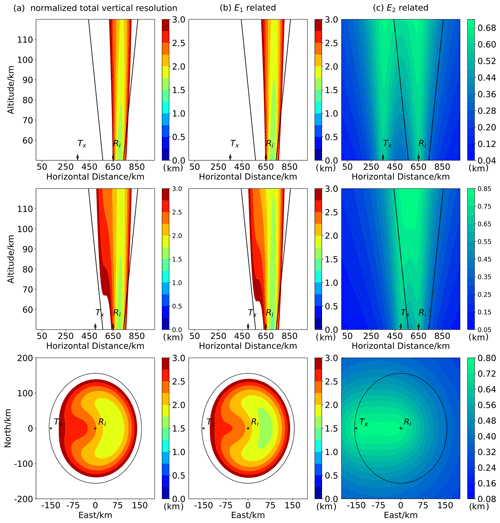Figure 7The normalized vertical resolution distribution using the analytical method described in this paper. The first and second rows represent a vertical section of height from 50 to 120 km. The third row represents the horizontal section at 90 km, and the receiving array is at the origin, with positive coordinate values representing the eastward or northward directions. The first row has the same parameters settings as Fig. 6 and is used to compare with Fig. 6. The E1-related resolution will change with the transmitter–receiver configuration because it considers the error term “δRs”. Thus, the total vertical resolution will change with the transmitter–receiver configuration. With the transmitter–receiver distance varying from 300 km (the first row) to 150 km (the second row), the total vertical resolution distribution is clearly changed. The third row is the perspective to the horizontal section at 90 km altitude for the second row. Normalized resolution values that exceed 3 km are not shown.

To get a perspective on the spatial-resolution distribution in three-dimensional space, Fig. 8 shows the normalized zonal, meridional and vertical spatial-resolution distributions for a multistatic radar link whose transmitter–receiver separation is 180 km and the transmitter is 30 south by east of the receiver. The classic monostatic meteor radar is a special case of a multistatic meteor radar system whose the baseline length is zero. By setting the transmitter–receiver distance to be zero in our program, a monostatic meteor radar's spatial resolution can also be obtained. In this case, the spatial-resolution distributions are highly symmetrical and correspond to the real characteristics of monostatic meteor radar (this is not shown here but can be found in Fig. S1 in the Supplement). In the discussion above, the receiver and transmitter antennas are all coplanar. By varying ${\mathit{\psi }}_{x}^{X,i}$, ${\mathit{\psi }}_{y}^{Y,i}$ and ${\mathit{\psi }}_{z}^{Z,i}$ in our program, non-coplanar receiver–transmitter antenna situations can also be studied. Slightly tilting the receiver horizontal plane (for example, set ${\mathit{\psi }}_{x}^{X,i}={\mathit{\psi }}_{y}^{Y,i}=\mathrm{5}{}^{\circ }$) causes the horizontal spatial distributions to change (see Figs. S2 and S3 in the Supplement). In practice, the Earth's curvature and local topography will lead to tilts in the receiver horizontal plane. This kind of tilt should be taken into account for multistatic meteor radar systems, and details relating to the parameter selections for this can be found in the Supplement.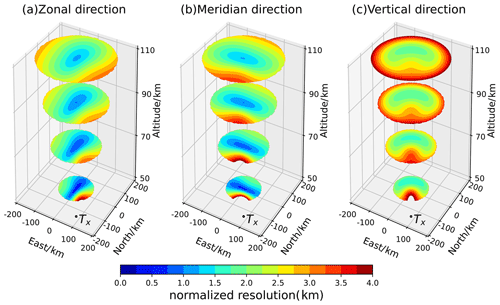Figure 8The 3D contour plot of the normalized resolution distribution for a multistatic radar link whose baseline length is 180 km and whose transmitter is 30 south by east of the receiver. The black dots represent the position right above the transmitter, and the receiving array is at the origin of the axes. Panels (a–c) are the normalized resolution distributions in the zonal, meridional and vertical directions, respectively. The subplot's four slice circles from bottom to top are the horizontal section at 50, 70, 90 and 110 km height, respectively. The region whose elevation angle of the receiver is less than 30 is not shown, and therefore the slice circles become larger from the bottom to the top. Normalized resolution values that exceed 4 km (which corresponds to 16 km resolution) are not shown.

The AoA error propagation process has been simplified to yield Eqs. (3) and (4) by using constant PDMEs. This is for the sake of providing the most general example of our method. If the analysis of AoA errors were to start from the original received voltage signals (e.g., Vaudrin et al., 2018), the error propagation process would depend on the specific receiver interferometer configuration and the specific signal processing method. The approach used here can be applied to different receiver antenna configurations or new signal processing algorithms. This would involve substitution of δ(ΔΨ1) and δ(ΔΨ2) into other mutually independent measuring errors to suit the experimental arrangement and then establishing a new AoA error propagation to obtain δθ and δϕ. This means rewriting the second and third term in Eq. (11) to the determine a new AoA error propagation matrix and new mutually independent measuring errors, respectively.

It worth noting that except for using the PDMEs as the start of the error propagation, all the analytical processes are built on mathematical error propagations. PDMEs include uncontrolled errors, such as those resulting from the returned wave being scattered from a few Fresnel zones along the meteor trail, phase calibration inaccuracy and noise. However, there are other error sources in practice. For example, aircraft or lightning interference and fading clutter from obstacles can cause further measurement errors in the AoA. These issues are related to actual physical situations and are beyond the scope of this text.

Knowing the valid observational volume for meteor detections and the errors associated with each detection is vital for a meteor radar system as it determines which meteors can be used to calculate wind velocities and also the uncertainties associated with the winds themselves. To reduce the influence of mutual antenna coupling or ground clutter, the elevation angle of a detection should be above a threshold, with 30 being typically used, and this sets the basic valid observational volume. Within this, the normalized vertical resolution varies, and in Figs. 7 and S4 in the Supplement only the areas of normalized vertical resolution with values below 3 km are shown, which we argue represents an acceptable sampling volume. In addition, as the transmitter–receiver distance increases, the sampling volume becomes smaller and the vertical resolution in this volume is reduced. This effect limits the practically usable transmitter–receiver distances for multistatic meteor radars.

The geometry of the multistatic meteor radar case also impacts on the ability of the radar to measure the Doppler shifts associated with drifting meteor trails within the observational volume. This is because the measured Doppler shift is produced by the component of the wind field in the direction of the Bragg vector, which in the multistatic configuration is divergent from the receiver's line of sight (see e.g., Spargo et al., 2019). The smaller the angle between the Bragg vector and the wind fields is, the larger the Doppler shift (and the higher the SNR) will be. This means that within the observational volume, the angular diversity of the Bragg vector should be taken into account in the wind retrieval process. A discussion of wind retrievals is beyond the scope of this text and will be considered in future work.

4 Conclusions

In this study, we have presented preliminary results from an analytical error method analysis of multistatic meteor radar system measurements of angles of arrival. The method can calculate the spatial resolution (the spatial uncertainty) in the zonal, meridional and vertical directions for an arbitrary receiving antenna array configuration in three-dimensional space. A given detected MTSP is located within the spatial-resolution volume with an equal probability. Higher values of spatial-resolution mean that this region needs more meteor counts or longer averaging to obtain a reliable accuracy. Our method shows that the spatial configuration of a multistatic system will greatly influence the spatial-resolution distribution in ENU coordinates and thus will in turn influence the retrieval accuracy of atmospheric parameters such as the wind field. The multistatic meteor radar system's spatial-resolution analysis is a key point in analyzing the accuracy of retrieved wind and other parameters. The influence of the spatial resolution on wind retrieval will be discussed in future work.

Appendix A

## A1 Coordinates rotation matrix

For a right-handed rectangular coordinate system XYZ, we rotate clockwise Ψx about the x axis to obtain a new coordinate. We specify that clockwise rotation satisfies the right-hand screw rule. A vector in XYZ, denoted as $\left(x,y,z{\right)}^{\mathrm{T}}$, is represented as $\left({x}^{\prime },{y}^{\prime },{z}^{\prime }{\right)}^{\mathrm{T}}$ in the new coordinate. The relationship between $\left(x,y,z{\right)}^{\mathrm{T}}$ and $\left({x}^{\prime },{y}^{\prime },{z}^{\prime }{\right)}^{\mathrm{T}}$ is as follows:

$\begin{array}{}\text{(A1)}& \left(\begin{array}{c}{x}^{\prime }\\ {y}^{\prime }\\ {z}^{\prime }\end{array}\right)={\mathbf{A}}_{\mathbf{x}}\left({\mathit{\psi }}_{x}\right)\left(\begin{array}{c}x\\ y\\ z\end{array}\right)=\left(\begin{array}{ccc}\mathrm{1}& \mathrm{0}& \mathrm{0}\\ \mathrm{0}& \mathrm{cos}{\mathit{\psi }}_{x}& \mathrm{sin}{\mathit{\psi }}_{x}\\ \mathrm{0}& -\mathrm{sin}{\mathit{\psi }}_{x}& \mathrm{cos}{\mathit{\psi }}_{x}\end{array}\right)\left(\begin{array}{c}x\\ y\\ z\end{array}\right).\end{array}$

Similarly, we rotate Ψy clockwise about the y axis to obtain a new coordinate. The presentation for a vector in new coordinates and the original can be linked by a matrix, Ay(ψy):

$\begin{array}{}\text{(A2)}& {\mathbf{A}}_{\mathbf{y}}\left({\mathit{\psi }}_{y}\right)=\left(\begin{array}{ccc}\mathrm{cos}{\mathit{\psi }}_{y}& \mathrm{0}& -\mathrm{sin}{\mathit{\psi }}_{y}\\ \mathrm{0}& \mathrm{1}& \mathrm{0}\\ \mathrm{sin}{\mathit{\psi }}_{y}& \mathrm{0}& \mathrm{cos}{\mathit{\psi }}_{y}\end{array}\right),\end{array}$

we rotate Ψz clockwise about the z axis to obtain a new coordinate. The presentation for a vector in new coordinates and original can be linked by a matrix Az(ψz):

$\begin{array}{}\text{(A3)}& {\mathbf{A}}_{\mathbf{z}}\left({\mathit{\psi }}_{z}\right)=\left(\begin{array}{ccc}\mathrm{cos}{\mathit{\psi }}_{z}& \mathrm{sin}{\mathit{\psi }}_{z}& \mathrm{0}\\ -\mathrm{sin}{\mathit{\psi }}_{z}& \mathrm{cos}{\mathit{\psi }}_{z}& \mathrm{0}\\ \mathrm{0}& \mathrm{0}& \mathrm{1}\end{array}\right).\end{array}$

For any two-coordinate systems XYZ and ${X}^{\prime }{Y}^{\prime }{Z}^{\prime }$ with co-origin, one can always rotate Ψx, Ψy and ψz clockwise in the order of the x, y and z axes, respectively, transforming XYZ to ${X}^{\prime }{Y}^{\prime }{Z}^{\prime }$ (Fig. A1). The presentation for a vector in ${X}^{\prime }{Y}^{\prime }{Z}^{\prime }$ and XYZ can be linked by a matrix, ${\mathbf{A}}_{\mathbf{R}}\left({\mathit{\psi }}_{x},{\mathit{\psi }}_{y},{\mathit{\psi }}_{z}\right)$.

$\begin{array}{}\text{(A4)}& \begin{array}{rl}& {\mathbf{A}}_{\mathbf{R}}\left({\mathit{\psi }}_{x},{\mathit{\psi }}_{y},{\mathit{\psi }}_{z}\right)={\mathbf{A}}_{\mathbf{z}}\left({\mathit{\psi }}_{z}\right){\mathbf{A}}_{\mathbf{y}}\left({\mathit{\psi }}_{y}\right){\mathbf{A}}_{\mathbf{x}}\left({\mathit{\psi }}_{x}\right)\\ & =\left(\begin{array}{cc}\mathrm{cos}{\mathit{\psi }}_{y}\mathrm{cos}{\mathit{\psi }}_{z}& \mathrm{sin}{\mathit{\psi }}_{x}\mathrm{sin}{\mathit{\psi }}_{y}\mathrm{cos}{\mathit{\psi }}_{z}+\mathrm{cos}{\mathit{\psi }}_{x}\mathrm{sin}{\mathit{\psi }}_{z}\\ -\mathrm{cos}{\mathit{\psi }}_{y}\mathrm{sin}{\mathit{\psi }}_{z}& -\mathrm{sin}{\mathit{\psi }}_{x}\mathrm{sin}{\mathit{\psi }}_{y}\mathrm{sin}{\mathit{\psi }}_{z}+\mathrm{cos}{\mathit{\psi }}_{x}\mathrm{cos}{\mathit{\psi }}_{z}\\ \mathrm{sin}{\mathit{\psi }}_{y}& -\mathrm{sin}{\mathit{\psi }}_{x}\mathrm{cos}{\mathit{\psi }}_{y}\end{array}\right\\ & \begin{array}{c}-\mathrm{cos}{\mathit{\psi }}_{x}\mathrm{sin}{\mathit{\psi }}_{y}\mathrm{cos}{\mathit{\psi }}_{z}+\mathrm{sin}{\mathit{\psi }}_{x}\mathrm{sin}{\mathit{\psi }}_{z}\\ \mathrm{cos}{\mathit{\psi }}_{x}\mathrm{sin}{\mathit{\psi }}_{y}\mathrm{sin}{\mathit{\psi }}_{z}+\mathrm{sin}{\mathit{\psi }}_{x}\mathrm{cos}{\mathit{\psi }}_{z}\\ \mathrm{cos}{\mathit{\psi }}_{x}\mathrm{cos}{\mathit{\psi }}_{y}\end{array})\end{array}\end{array}$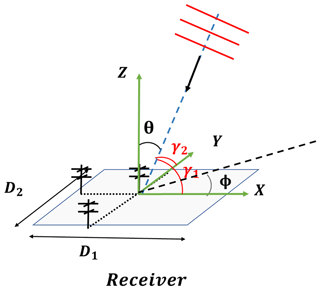Figure A2The receiving array geometry (only three antennas are shown for clarity).

We call ${\mathbf{A}}_{\mathbf{R}}\left({\mathit{\psi }}_{x},{\mathit{\psi }}_{y},{\mathit{\psi }}_{z}\right)$ the coordinate rotation matrix.

## A2 AoA measuring errors

In coordinate XYZ, AoAs includes zenith angle θ and azimuth angle ϕ. In the plane wave approximation, the radio wave is at angle γ1 and γ2 with an antenna array (Fig. A2). There is a phase difference ΔΨ1 and ΔΨ2 between two antennas (Fig. 1). ΔΨ1 and ΔΨ2 can be expressed as follows:

$\begin{array}{}\text{(A5)}& & \mathrm{\Delta }{\mathrm{\Psi }}_{\mathrm{1}}=\frac{\mathrm{2}\mathit{\pi }{D}_{\mathrm{1}}\mathrm{cos}{\mathit{\gamma }}_{\mathrm{1}}}{\mathit{\lambda }},\text{(A6)}& & \mathrm{\Delta }{\mathrm{\Psi }}_{\mathrm{2}}=\frac{\mathrm{2}\mathit{\pi }{D}_{\mathrm{2}}\mathrm{cos}{\mathit{\gamma }}_{\mathrm{2}}}{\mathit{\lambda }}.\end{array}$

Using γ1, γ2 the AoA can be expressed as follows:

$\begin{array}{}\text{(A7)}& & {\mathrm{cos}}^{\mathrm{2}}{\mathit{\gamma }}_{\mathrm{1}}+{\mathrm{cos}}^{\mathrm{2}}{\mathit{\gamma }}_{\mathrm{2}}+{\mathrm{cos}}^{\mathrm{2}}\mathit{\theta }=\mathrm{1},\text{(A8)}& & \mathrm{tan}\mathit{\varphi }=\frac{\mathrm{cos}{\mathit{\gamma }}_{\mathrm{2}}}{\mathrm{cos}{\mathit{\gamma }}_{\mathrm{1}}},\end{array}$

or in another expression:

$\begin{array}{}\text{(A9)}& & \mathrm{cos}{\mathit{\gamma }}_{\mathrm{1}}=\mathrm{sin}\mathit{\theta }\mathrm{cos}\mathit{\varphi },\text{(A10)}& & \mathrm{cos}{\mathit{\gamma }}_{\mathrm{2}}=\mathrm{sin}\mathit{\theta }\mathrm{sin}\mathit{\varphi },\end{array}$

substitute cos γ1 and cos γ2 in Eqs. (A7) and (A8) by using Eqs. (A5) and (A6):

$\begin{array}{}\text{(A11)}& & {\mathrm{cos}}^{\mathrm{2}}\mathit{\theta }=\mathrm{1}-{\left(\frac{\mathit{\lambda }}{\mathrm{2}\mathit{\pi }}\right)}^{\mathrm{2}}\left(\frac{{\mathrm{\Delta }}^{\mathrm{2}}{\mathrm{\Psi }}_{\mathrm{1}}}{{D}_{\mathrm{1}}^{\mathrm{2}}}+\frac{{\mathrm{\Delta }}^{\mathrm{2}}{\mathrm{\Psi }}_{\mathrm{2}}}{{D}_{\mathrm{2}}^{\mathrm{2}}}\right),\text{(A12)}& & \mathrm{ln}\left(\mathrm{tan}\mathit{\varphi }\right)=\mathrm{ln}\left({D}_{\mathrm{1}}\mathrm{\Delta }{\mathrm{\Psi }}_{\mathrm{2}}\right)-\mathrm{ln}\left({D}_{\mathrm{2}}\mathrm{\Delta }{\mathrm{\Psi }}_{\mathrm{1}}\right).\end{array}$

Equations (A11) and (A12) link the phase difference with the AoA and expanding θ and ϕ, ΔΨ1 and ΔΨ2 to the first order.

$\begin{array}{}\text{(A13)}& \begin{array}{rl}& \mathrm{2}\mathrm{cos}\mathit{\theta }\mathrm{sin}\mathit{\theta }\mathit{\delta }\mathit{\theta }\\ & ={\left(\frac{\mathit{\lambda }}{\mathrm{2}\mathit{\pi }}\right)}^{\mathrm{2}}\cdot \left[\frac{\mathrm{2}\mathrm{\Delta }{\mathrm{\Psi }}_{\mathrm{1}}\mathit{\delta }\left(\mathrm{\Delta }{\mathrm{\Psi }}_{\mathrm{1}}\right)}{{D}_{\mathrm{1}}^{\mathrm{2}}}+\frac{\mathrm{2}\mathrm{\Delta }{\mathrm{\Psi }}_{\mathrm{2}}\mathit{\delta }\left(\mathrm{\Delta }{\mathrm{\Psi }}_{\mathrm{2}}\right)}{{D}_{\mathrm{2}}^{\mathrm{2}}}\right]\end{array}\text{(A14)}& \mathit{\delta }\mathit{\varphi }=\frac{\mathrm{sin}\mathit{\varphi }\mathrm{cos}\mathit{\varphi }}{\mathrm{\Delta }{\mathrm{\Psi }}_{\mathrm{2}}}\mathit{\delta }\left(\mathrm{\Delta }{\mathrm{\Psi }}_{\mathrm{2}}\right)-\frac{\mathrm{sin}\mathit{\varphi }\mathrm{cos}\mathit{\varphi }}{\mathrm{\Delta }{\mathrm{\Psi }}_{\mathrm{1}}}\mathit{\delta }\left(\mathrm{\Delta }{\mathrm{\Psi }}_{\mathrm{1}}\right)\end{array}$

For Eqs. (A13) and (A14), substitute ΔΨ1 and ΔΨ2 using Eqs. (A5), (A6) and (A9), (A10) to the functions of θ, ϕ. Now Eqs. (3) and (4) have been proven. If the zenith angle $\mathit{\theta }=\mathrm{0}{}^{\circ }$, we stipulate that $\frac{\mathrm{cos}\mathit{\varphi }}{\mathrm{sin}\mathit{\theta }}$ and $\frac{\mathrm{sin}\mathit{\varphi }}{\mathrm{sin}\mathit{\theta }}$ are 1.

## A3 Radial distance measuring error

Expanding Rs, R and cos α in Eq. (1) to the first order, δRs can be expressed as a function of δR and δ(cos α):

$\begin{array}{}\text{(A15)}& \begin{array}{rl}\mathit{\delta }{R}_{\mathrm{s}}& =\frac{{R}^{\mathrm{2}}-\mathrm{2}Rd\mathrm{cos}\mathit{\alpha }+{d}^{\mathrm{2}}}{\mathrm{2}\left(R-d\mathrm{cos}\mathit{\alpha }{\right)}^{\mathrm{2}}}\mathit{\delta }R\\ & \phantom{\rule{0.25em}{0ex}}+\frac{d\left({R}^{\mathrm{2}}-{d}^{\mathrm{2}}\right)}{\mathrm{2}\left(R-d\mathrm{cos}\mathit{\alpha }{\right)}^{\mathrm{2}}}\mathit{\delta }\left(\mathrm{cos}\mathit{\alpha }\right),\end{array}\end{array}$

where α is the angle between Rs and the Xi axis. We denote the zenith and azimuth angles in coordinate XiYiZi as θ and ϕ, respectively. The relationship between α and θ, i.e., ϕ, is

$\begin{array}{}\text{(A16)}& \mathrm{cos}\mathit{\alpha }=\mathrm{sin}{\mathit{\theta }}^{\prime }\mathrm{cos}{\mathit{\varphi }}^{\prime }.\end{array}$

Using the coordinate rotation matrix ${\mathbf{A}}_{\mathbf{R}}\left({\mathit{\psi }}_{x}^{X,i},{\mathit{\psi }}_{y}^{Y,i},{\mathit{\psi }}_{z}^{Z,i}\right)$ and $\mathrm{sin}{\mathit{\theta }}^{\prime }\mathrm{cos}{\mathit{\varphi }}^{\prime }$ can be expressed as the function of AoA:

$\begin{array}{}\text{(A17)}& \begin{array}{rl}\mathrm{sin}{\mathit{\theta }}^{\prime }\mathrm{cos}{\mathit{\varphi }}^{\prime }& ={A}_{\mathrm{11}}\mathrm{sin}\mathit{\theta }\mathrm{cos}\mathit{\varphi }+{A}_{\mathrm{12}}\mathrm{sin}\mathit{\theta }\mathrm{sin}\mathit{\varphi }\\ & \phantom{\rule{0.25em}{0ex}}+{A}_{\mathrm{13}}\mathrm{cos}\mathit{\theta }.\end{array}\end{array}$

Aij represent the elements in matrix ${\mathbf{A}}_{\mathbf{R}}\left({\mathit{\psi }}_{x}^{X,i},{\mathit{\psi }}_{y}^{Y,i},{\mathit{\psi }}_{z}^{Z,i}$) for $i,j=\mathrm{1},\mathrm{2},\mathrm{3}$.

Using Eqs. (A16) and (A17), δ(cos α) can be expressed as a function of δθ and δϕ as follows:

$\begin{array}{}\text{(A18)}& \begin{array}{rl}& \mathit{\delta }\left(\mathrm{cos}\mathit{\alpha }\right)\\ & =\left({A}_{\mathrm{11}}\mathrm{cos}\mathit{\theta }\mathrm{cos}\mathit{\varphi }+{A}_{\mathrm{12}}\mathrm{cos}\mathit{\theta }\mathrm{sin}\mathit{\varphi }-{A}_{\mathrm{13}}\mathrm{sin}\mathit{\theta }\right)\mathit{\delta }\mathit{\theta }\\ & +\left(-{A}_{\mathrm{11}}\mathrm{sin}\mathit{\theta }\mathrm{sin}\mathit{\varphi }+{A}_{\mathrm{12}}\mathrm{sin}\mathit{\theta }\mathrm{cos}\mathit{\varphi }\right)\mathit{\delta }\mathit{\varphi }.\end{array}\end{array}$

Finally, δRs can be expressed as the function of $\mathit{\delta }R,\mathit{\delta }\mathit{\theta },\mathit{\delta }\mathit{\varphi }$ as follows.

$\begin{array}{}\text{(A19)}& \begin{array}{rl}\mathit{\delta }{R}_{\mathrm{s}}=& \phantom{\rule{0.25em}{0ex}}F\left(\mathit{\delta }R,\mathit{\delta }\mathit{\theta },\mathit{\delta }\mathit{\varphi }\right)={f}_{R}\left(\mathit{\theta },\mathit{\varphi }\right)\mathit{\delta }R+{f}_{\mathit{\theta }}\left(\mathit{\theta },\mathit{\varphi }\right)\mathit{\delta }\mathit{\theta }\\ & +{f}_{\mathit{\varphi }}\left(\mathit{\theta },\mathit{\varphi }\right)\mathit{\delta }\mathit{\varphi }\end{array}\end{array}$
$\begin{array}{}\text{(A20)}& \begin{array}{rl}& {f}_{R}\left(\mathit{\theta },\mathit{\varphi }\right)=\\ & \frac{{d}^{\mathrm{2}}+{R}^{\mathrm{2}}-\mathrm{2}Rd\left({A}_{\mathrm{11}}\mathrm{sin}\mathit{\theta }\mathrm{cos}\mathit{\varphi }+{A}_{\mathrm{12}}\mathrm{sin}\mathit{\theta }\mathrm{sin}\mathit{\varphi }+{A}_{\mathrm{13}}\mathrm{cos}\mathit{\theta }\right)}{\mathrm{2}{\left[R-d\left({A}_{\mathrm{11}}\mathrm{sin}\mathit{\theta }\mathrm{cos}\mathit{\varphi }+{A}_{\mathrm{12}}\mathrm{sin}\mathit{\theta }\mathrm{sin}\mathit{\varphi }+{A}_{\mathrm{13}}\mathrm{cos}\mathit{\theta }\right)\right]}^{\mathrm{2}}}\end{array}\end{array}$
$\begin{array}{}\text{(A21)}& \begin{array}{rl}& {f}_{\mathit{\theta }}\left(\mathit{\theta },\mathit{\varphi }\right)=\\ & \frac{d\left({R}^{\mathrm{2}}-{d}^{\mathrm{2}}\right)\left({A}_{\mathrm{11}}\mathrm{cos}\mathit{\theta }\mathrm{cos}\mathit{\varphi }+{A}_{\mathrm{12}}\mathrm{cos}\mathit{\theta }\mathrm{sin}\mathit{\varphi }-{A}_{\mathrm{13}}\mathrm{sin}\mathit{\theta }\right)}{\mathrm{2}{\left[R-d\left({A}_{\mathrm{11}}\mathrm{sin}\mathit{\theta }\mathrm{cos}\mathit{\varphi }+{A}_{\mathrm{12}}\mathrm{sin}\mathit{\theta }\mathrm{sin}\mathit{\varphi }+{A}_{\mathrm{13}}\mathrm{cos}\mathit{\theta }\right)\right]}^{\mathrm{2}}}\end{array}\end{array}$
$\begin{array}{}\text{(A22)}& \begin{array}{rl}& {f}_{\mathit{\varphi }}\left(\mathit{\theta },\mathit{\varphi }\right)=\\ & \frac{d\left({R}^{\mathrm{2}}-{d}^{\mathrm{2}}\right)\left(-{A}_{\mathrm{11}}\mathrm{sin}\mathit{\theta }\mathrm{sin}\mathit{\varphi }+{A}_{\mathrm{12}}\mathrm{sin}\mathit{\theta }\mathrm{cos}\mathit{\varphi }\right)}{\mathrm{2}{\left[R-d\left({A}_{\mathrm{11}}\mathrm{sin}\mathit{\theta }\mathrm{cos}\mathit{\varphi }+{A}_{\mathrm{12}}\mathrm{sin}\mathit{\theta }\mathrm{sin}\mathit{\varphi }+{A}_{\mathrm{13}}\mathrm{cos}\mathit{\theta }\right)\right]}^{\mathrm{2}}}\end{array}\end{array}$

## A4 True error of E2

The total length of side AC and side AB represents the pulse width. Side AC equals side CB and they are both equal to half of the pulse width S. In XiYiZi, the presentation of point A is $\left({x}_{i},{y}_{i},{z}_{i}\right)$, the receiver is (0,0,0) and Ti is (d,0,0). The distance between Ti and A is ${R}_{i}=R-{R}_{\mathrm{s}}$. We denote the presentation of point B and C in XiYiZi as $\left({x}_{B},{y}_{B},{z}_{B}\right)$ and $\left({x}_{C},{y}_{C},{z}_{C}\right)$, respectively. We use vector collinear to establish equations for B and C. Therefore, one can obtain the coordinates of point B and C by the following equations:

$\begin{array}{}\text{(A23)}& \left({x}_{B},{y}_{B},{z}_{B}{\right)}^{\mathrm{T}}=\frac{{R}_{\mathrm{s}}-S}{{R}_{\mathrm{s}}}\left({x}_{i},{y}_{i},{z}_{i}{\right)}^{\mathrm{T}},\end{array}$

$\begin{array}{}\text{(A24)}& \left({x}_{C}-d,{y}_{C},{z}_{C}{\right)}^{\mathrm{T}}=\frac{{R}_{i}-S}{{R}_{i}}\left({x}_{i}-d,{y}_{i},{z}_{i}{\right)}^{\mathrm{T}}.\end{array}$

For isosceles triangle ABC, the perpendicular line AD intersects side CB at the midpoint D. Thus, we obtain the coordinate value of D in XiYiZi as follows:

$\begin{array}{}\text{(A25)}& \begin{array}{rl}\left({x}_{D},{y}_{D},{z}_{D}\right)=& \phantom{\rule{0.25em}{0ex}}\frac{\mathrm{1}}{\mathrm{2}}\left({x}_{B}+{x}_{c},{y}_{B}+{y}_{c},{z}_{b}+{z}_{c}\right)\\ =& \phantom{\rule{0.25em}{0ex}}\frac{\mathrm{1}}{\mathrm{2}}\left(\left({a}_{\mathrm{1}}+{a}_{\mathrm{2}}\right){x}_{i}-{a}_{\mathrm{2}}d\\ & +d,\left({a}_{\mathrm{1}}+{a}_{\mathrm{2}}\right),{y}_{i}\left({a}_{\mathrm{1}}+{a}_{\mathrm{2}}\right){z}_{i}\right).\end{array}\end{array}$

We denote ${a}_{\mathrm{1}}=\frac{{R}_{\mathrm{s}}-S}{{R}_{\mathrm{s}}}$, ${a}_{\mathrm{2}}=\frac{{R}_{i}-S}{{R}_{i}}$. Finally, one can obtain the error vector of E2 as vector DA in XiYiZi:

$\begin{array}{}\text{(A26)}& \begin{array}{rl}\mathbit{D}\mathbit{A}=\left(& \frac{\left(\mathrm{2}-{a}_{\mathrm{1}}-{a}_{\mathrm{2}}\right){x}_{i}+d\left({a}_{\mathrm{2}}-\mathrm{1}\right)}{\mathrm{2}},\frac{\mathrm{2}-{a}_{\mathrm{1}}-{a}_{\mathrm{2}}}{\mathrm{2}}{y}_{i},\\ & \phantom{\rule{0.25em}{0ex}}\frac{\mathrm{2}-{a}_{\mathrm{1}}-{a}_{\mathrm{2}}}{\mathrm{2}}{z}_{i}{\right)}^{\mathrm{T}}.\end{array}\end{array}$

## A5 Calculate AoA and range information in XiYiZi

For a space point $\left({x}_{i},{y}_{i},{z}_{i}\right)$ in XiYiZi, which represents a MTSP, Rs can be solved easily as follows:

$\begin{array}{}\text{(A27)}& \begin{array}{rl}& {R}_{\mathrm{s}}=\left({x}_{i},{y}_{i},{z}_{i}\right)\\ & {R}_{\mathrm{s}}=\sqrt{{x}_{i}^{\mathrm{2}}+{y}_{i}^{\mathrm{2}}+{z}_{i}^{\mathrm{2}}}.\end{array}\end{array}$

The distance between transmitter Ti and receiver RX is di, as shown in Fig. 4a. Thus, the coordinate value of Ti in XiYiZi is $\left({d}_{i},\mathrm{0},\mathrm{0}\right)$, and Ri can be solved as follows:

$\begin{array}{}\text{(A28)}& {R}_{i}=\sqrt{\left({x}_{i}-{d}_{i}{\right)}^{\mathrm{2}}+{y}_{i}^{\mathrm{2}}+{z}_{i}^{\mathrm{2}}}.\end{array}$

Before we calculate the AoAs in XiYiZi, the representation of unit vectors of the x, y and z axes in XiYiZi needs to be known. In XYZ those unit vectors are easily represented as $\left(\mathrm{1},\mathrm{0},\mathrm{0}{\right)}^{\mathrm{T}}$, $\left(\mathrm{0},\mathrm{1},\mathrm{0}{\right)}^{\mathrm{T}}$, $\left(\mathrm{0},\mathrm{0},\mathrm{1}{\right)}^{\mathrm{T}}$. Through the coordinate rotation matrix ${\mathbf{A}}_{\mathbf{R}}\left({\mathit{\psi }}_{x}^{X,i},{\mathit{\psi }}_{y}^{Y,i}$,${\mathit{\psi }}_{z}^{Z,i}\right)$, one can get those unit vector's representation in XiYiZi as follows:

$\begin{array}{}\text{(A29)}& \begin{array}{r}{\mathbit{n}}_{\mathbit{x}}=\left({A}_{\mathrm{11}},{A}_{\mathrm{21}},{A}_{\mathrm{31}}{\right)}^{\mathrm{T}}\\ {\mathbit{n}}_{\mathbit{y}}=\left({A}_{\mathrm{12}},{A}_{\mathrm{22}},{A}_{\mathrm{32}}{\right)}^{\mathrm{T}}\\ {\mathbit{n}}_{\mathbit{z}}=\left({A}_{\mathrm{13}},{A}_{\mathrm{23}},{A}_{\mathrm{33}}{\right)}^{\mathrm{T}}.\end{array}\end{array}$

nx, ny and nz are unit vectors of the x, y and z axes, respectively, and Aij is the element's 3×3 matrix ${\mathbf{A}}_{\mathbf{R}}\left({\mathit{\psi }}_{x}^{X,i},{\mathit{\psi }}_{y}^{Y,i}$,${\mathit{\psi }}_{z}^{Z,i}$) for $i,j=\mathrm{1},\mathrm{2},\mathrm{3}$. Now the AoA can be obtained as follows:

$\begin{array}{}\text{(A30)}& & \mathrm{cos}\mathit{\theta }=\frac{{\mathbit{R}}_{\mathbf{s}}}{{R}_{\mathrm{s}}}\cdot {\mathbit{n}}_{\mathbit{z}},\text{(A31)}& & \mathrm{sin}\mathit{\theta }=\sqrt{\mathrm{1}-{\mathrm{cos}}^{\mathrm{2}}\mathit{\theta }},\text{(A32)}& & \mathrm{cos}\mathit{\varphi }=\frac{{\mathbit{R}}_{\mathbf{s}}}{{R}_{\mathrm{s}}}\cdot \frac{{\mathbit{n}}_{\mathbit{x}}}{\mathrm{sin}\mathit{\theta }},\text{(A33)}& & \mathrm{sin}\mathit{\varphi }=\frac{{\mathbit{R}}_{\mathbf{s}}}{{R}_{\mathrm{s}}}\cdot \frac{{\mathbit{n}}_{\mathbit{y}}}{\mathrm{sin}\mathit{\theta }},\end{array}$

for $\mathrm{0}{}^{\circ }<\mathit{\theta }<\mathrm{180}{}^{\circ }$ and $\mathrm{0}{}^{\circ }\le \mathit{\varphi }<\mathrm{360}{}^{\circ }$. When $\mathit{\theta }=\mathrm{0}{}^{\circ }$, we handle it the way as same as in Sect. A2.

Code availability

The program to calculate the 3D spatial resolution distributions is available at https://doi.org/10.17605/OSF.IO/2AMXC (Zhong, 2021).

Data availability

Supplement

Author contributions

WZ, XX and WY designed the study. WZ deduced the formulas and wrote the program. WZ wrote the paper for the first version. XX supervised the work and provided valuable comments. IMR revised the paper. All of the authors discussed the results and commented on the paper.

Competing interests

The authors declare that they have no conflict of interest.

Acknowledgements

Wei Zhong thanks Jia Mingjiao for useful discussions and Zeng Jie for checking the equations in the manuscript.

Financial support

This research has been supported by the B-type Strategic Priority Program of CAS Grant (grant no. XDB41000000), the National Natural Science Foundation of China (grant nos. 41774158, 41974174, 41831071, and 41904135), the CNSA pre-research Project on Civil Aerospace Technologies (grant no. D020105), the Fundamental Research Funds for the Central Universities (grant no. YD3420002004), the Joint Open Fund of Mengcheng National Geophysical Observatory (grant no. MENGO‐202009), and the Open Research Project of Large Research Infrastructures of CAS “Study on the interaction between low/mid-latitude atmosphere and ionosphere based on the Chinese Meridian Project”.

Review statement

This paper was edited by Markus Rapp and reviewed by two anonymous referees.

References

Browning, K. A. and Wexler, R.: The Determination of Kinematic Properties of a Wind Field Using Doppler Radar, J. Appl. Meteorol., 7, 105–113, https://doi.org/10.1175/1520-0450(1968)007<0105:Tdokpo>2.0.Co;2, 1968.

Ceplecha, Z., Borovička, J., Elford, W. G., ReVelle, D. O., Hawkes, R. L., Porubčan, V., and Šimek, M.: Meteor Phenomena and Bodies, Space Sci. Rev., 84, 327–471, https://doi.org/10.1023/A:1005069928850, 1998.

Chau, J. L., Stober, G., Hall, C. M., Tsutsumi, M., Laskar, F. I., and Hoffmann, P.: Polar mesospheric horizontal divergence and relative vorticity measurements using multiple specular meteor radars, Radio Sci., 52, 811–828, https://doi.org/10.1002/2016rs006225, 2017.

Chau, J. L., Urco, J. M., Vierinen, J. P., Volz, R. A., Clahsen, M., Pfeffer, N., and Trautner, J.: Novel specular meteor radar systems using coherent MIMO techniques to study the mesosphere and lower thermosphere, Atmos. Meas. Tech., 12, 2113–2127, https://doi.org/10.5194/amt-12-2113-2019, 2019.

Dorey, J., Blanchard, Y., and Christophe, F.: Le projet RIAS, une approche nouvelle du radar de surveillance aérienne, Onde Electr., 64, 15–20, 1984.

Hocking, W. K.: Spatial distribution of errors associated with multistatic meteor radar, Earth Planets Space, 70, 93, https://doi.org/10.1186/s40623-018-0860-2, 2018.

Hocking, W. K., Fuller, B., and Vandepeer, B.: Real-time determination of meteor-related parameters utilizing modem digital technology, J. Atmos. Sol.-Terr. Phy., 63, 155–169, https://doi.org/10.1016/s1364-6826(00)00138-3, 2001.

Holdsworth, D. A., Reid, I. M., and Cervera, M. A.: Buckland Park all-sky interferometric meteor radar, Radio Sci., 39, RS5009, https://doi.org/10.1029/2003rs003014, 2004.

Jacobi, C., Hoffmann, P., and Kürschner, D.: Trends in MLT region winds and planetary waves, Collm (52 N, 15 E), Ann. Geophys., 26, 1221–1232, https://doi.org/10.5194/angeo-26-1221-2008, 2008.

Jia, M. J., Xue, X. H., Dou, X. K., Tang, Y. H., Yu, C., Wu, J. F., Xu, J. Y., Yang, G. T., Ning, B. Q., and Hoffmann, L.: A case study of A mesoscale gravity wave in the MLT region using simultaneous multi-instruments in Beijing, J. Atmos. Sol.-Terr. Phy., 140, 1–9, https://doi.org/10.1016/j.jastp.2016.01.007, 2016.

Jones, J., Webster, A. R., and Hocking, W. K.: An improved interferometer design for use with meteor radars, Radio Sci., 33, 55–65, https://doi.org/10.1029/97rs03050, 1998.

Kang, C.: Meteor radar signal processing and error analysis, Dissertations & Theses – Gradworks, Department of Aerospace Engineering and Sciences, University of Colorado, US, 2008.

Philippe, W. and Corbin, H.: On the Analysis of Single-Doppler Radar Data, J. Appl. Meteorol., 18, 532–542, https://doi.org/10.1175/1520-0450(1979)018<0532:OTAOSD>2.0.CO;2, 1979.

Reid, I. M.: Some aspects of Doppler radar measurements of the mean and fluctuating components of the wind-field in the upper middle atmosphere, J. Atmos. Terr. Phys., 49, 467–484, https://doi.org/10.1016/0021-9169(87)90041-9, 1987.

Reid, I. M.: MF and HF Spaced Antenna radar techniques for investigating the dynamics and structure of the 50 to 110 km height region: A review, Progress in Earth and Planetary Science, 2, 33, https://doi.org/10.1186/s40645-015-0060-7, 2015.

Spargo, A. J., Reid, I. M., and MacKinnon, A. D.: Multistatic meteor radar observations of gravity-wave–tidal interaction over southern Australia, Atmos. Meas. Tech., 12, 4791–4812, https://doi.org/10.5194/amt-12-4791-2019, 2019.

Stober, G., Sommer, S., Rapp, M., and Latteck, R.: Investigation of gravity waves using horizontally resolved radial velocity measurements, Atmos. Meas. Tech., 6, 2893–2905, https://doi.org/10.5194/amt-6-2893-2013, 2013.

Stober, G. and Chau, J. L.: A multistatic and multifrequency novel approach for specular meteor radars to improve wind measurements in the MLT region, Radio Sci., 50, 431–442, https://doi.org/10.1002/2014rs005591, 2015.

Stober, G., Chau, J. L., Vierinen, J., Jacobi, C., and Wilhelm, S.: Retrieving horizontally resolved wind fields using multi-static meteor radar observations, Atmos. Meas. Tech., 11, 4891–4907, https://doi.org/10.5194/amt-11-4891-2018, 2018.

Urco, J. M., Chau, J. L., Weber, T., Vierinen, J. P., and Volz, R.: Sparse Signal Recovery in MIMO Specular Meteor Radars With Waveform Diversity, IEEE T. Geosci. Remote, 57, 10088–10098, https://doi.org/10.1109/tgrs.2019.2931375, 2019.

Vaudrin, C. V., Palo, S. E., and Chau, J. L.: Complex Plane Specular Meteor Radar Interferometry, Radio Sci., 53, 112–128, https://doi.org/10.1002/2017rs006317, 2018.

Vierinen, J., Chau, J. L., Pfeffer, N., Clahsen, M., and Stober, G.: Coded continuous wave meteor radar, Atmos. Meas. Tech., 9, 829–839, https://doi.org/10.5194/amt-9-829-2016, 2016.

Vierinen, J., Chau, J. L., Charuvil, H., Urco, J. M., Clahsen, M., Avsarkisov, V., Marino, R., and Volz, R.: Observing Mesospheric Turbulence With Specular Meteor Radars: A Novel Method for Estimating Second-Order Statistics of Wind Velocity, Earth and Space Science, 6, 1171–1195, https://doi.org/10.1029/2019ea000570, 2019.

Wilhelm, S., Stober, G., and Chau, J. L.: A comparison of 11-year mesospheric and lower thermospheric winds determined by meteor and MF radar at 69  N, Ann. Geophys., 35, 893–906, https://doi.org/10.5194/angeo-35-893-2017, 2017.

Xue, X. H., Dou, X. K., Lei, J., Chen, J. S., Ding, Z. H., Li, T., Gao, Q., Tang, W. W., Cheng, X. W., and Wei, K.: Lower thermospheric-enhanced sodium layers observed at low latitude and possible formation: Case studies, J. Geophys. Res.-Space, 118, 2409–2418, https://doi.org/10.1002/jgra.50200, 2013.

Yi, W., Xue, X. H., Reid, I. M., Younger, J. P., Chen, J. S., Chen, T. D., and Li, N.: Estimation of Mesospheric Densities at Low Latitudes Using the Kunming Meteor Radar Together With SABER Temperatures, J. Geophys. Res.-Space, 123, 3183–3195, https://doi.org/10.1002/2017ja025059, 2018.

Younger, J. P. and Reid, I. M.: Interferometer angle-of-arrival determination using precalculated phases, Radio Sci., 52, 1058–1066, https://doi.org/10.1002/2017rs006284, 2017.

Zhong, W.: 3D Multistatic Meteor Radar Error Anlanyze, OSF [code], https://doi.org/10.17605/OSF.IO/2AMXC, 2021.